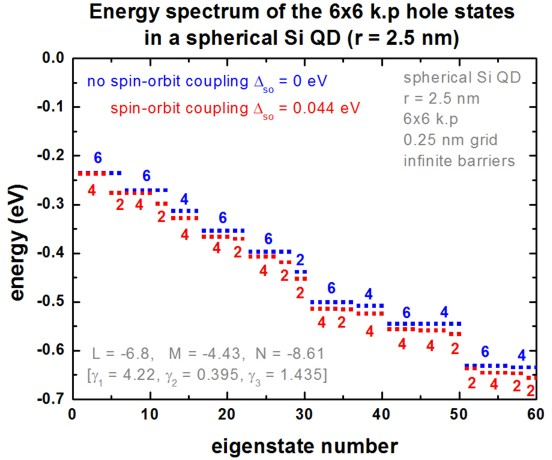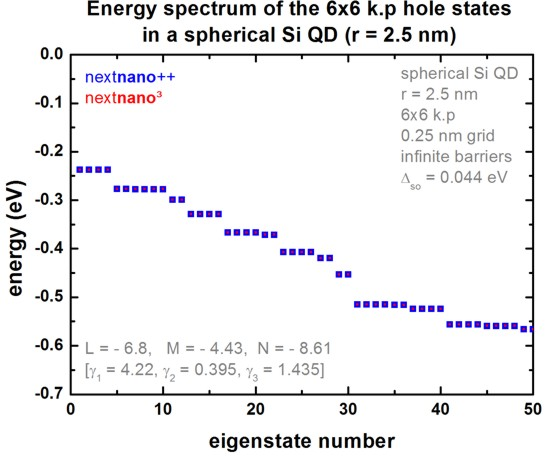nextnano.com  GUI: nextnanomat  Tool: nextnano++  Tool: nextnano³  Tool: nextnano.QCL  Download | Search | Copyright | News | Publications  * password protected nextnano³ software3D QD 6-band k.p

# nextnano3 - Tutorial

## Hole energy levels of an "artificial atom" - Spherical Si Quantum Dot (6-band k.p)

Author: Stefan Birner

```-> 3DsphericSiQD_d5nm_6bandkp_nn3.in / *_nnp.in - ```spherical Si QD with diameter 5 nm``` - ```input file for the nextnano3 and nextnano++ software

`==> ` Download these input files
`    `If you don't have a password yet, you have to first sign up for a free evaluation license in order to download these input files.```  ```

## Hole energy levels of an "artificial atom" - Spherical Si Quantum Dot (6-band k.p)

```-> 3DsphericSiQD_d5nm_6bandkp_nn3.in - ```spherical Si QD with diameter 5 nm

Here, we want to calculate the energy spectrum of a spherical Si quantum dot of radius 2.5 nm.

We assume that the barriers at the QD boundaries are infinite.
The potential inside the QD is assumed to be 0 eV.
We use a grid resolution of 0.25 nm.
We solve the 6-band k.p Schrödinger equation for the hole eigenstates.

The following 6-band k.p parameters are used:
```    L = -6.8           ! ```[Burdov] V.A. Burdov, JETP 94, 411 (2002)```    M = -4.43          ! ```[Burdov])```    N = -8.61          ! ```[Burdov])
`   Deltaso = 0.044 eV`

``` 6x6kp-parameters = -6.8d0 -4.43d0 -8.61d0 ! L, M, N [hbar^2/2m]                      0.044d0               ! Deltasplit-off [eV]```   spin-orbit splitting energy``` ```These L, M, N parameters correspond to the following Luttinger parameters:
```   gamma1 = 4.22    gamma2 = 0.395    gamma3 = 1.435  ```

The following figure shows the hole eigenenergy spectrum of the Si QD (diameter = 5 nm) calculated with a 6-band k.p Hamiltonian.For comparison, we also display the energy spectrum where we assumed zero spin-orbit splitting energy.
In this case there is a six-fold symmetry. Spin-orbit splitting reduces this degeneracy to 4 and 2.
In general, each state is two-fold degenerate due to spin.

(Note: We used a cuboidal shaped quantum cluster although we could have used a spherical quantum cluster to reduce the size of the 6-band k.p Hamiltonian matrix that has to be solved for the eigenstates.
If m grid points can be excluded from the quantum region due to an optimal choice of quantum region shape, the dimension N of the 6-band k.p matrix is reduced by N`-`6m.)

Following the paper of [Burdov], one can calculate the ground state energy for this particular system from the L and M parameters:

E1 = `-` hbar2 pi2 / ( 2 mh R2 ) = -0.314 eV    (using mh = 0.192 m0 as [Burdov] where he uses incorrect k.p parameters: In his definition L must be `-`5.8 and M = `-`3.43.)
E1 = `-` hbar2 pi2 / ( 2 mh R2 ) = -0.254 eV    (using mh = 0.237 m0 as V.A. Belyakov, V.A. Burdov, J. Phys.: Condens. Matter 20, 025213 (2008))
The latter is in much better agreement to our calculations.

mh is given by:
mh = 3 m0 / (  L      + 2  M    ) = 3 m0 / ( `-`6.8 + 2 * (`-`4.43)) = `-`0.192 m0     [Burdov]
mh = 3 m0 / ( (L+1) + 2 (M+1)) = 3 m0 / ( `-`5.8 + 2 * (`-`3.43)) = `-`0.237 m0    [Belyakov/Burdov]
The latter definition is consistent to our implementation of the k.p Hamiltonian.

The discrepancy of these equations arises because there are two different definitions of the L,M parameters available in the literature.
More details...

### nextnano³ vs. nextnano++ comparison

The following figure compares the nextnano³ results with the nextnano++ results.
The results of both simulators are in excellent agreement.In our case, the '`chearn`' eigenvalue solver (Arnoldi method that uses Chebyshev polynomials as preconditioner) missed some degenerate eigenvalues. So probably one has to adjust some eigenvalue solver parameters to increase the accuracy.
• Please help us to improve our tutorial. Send comments to``` support [at] nextnano.com```.